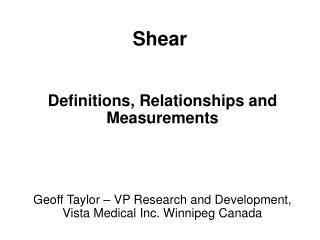DownloadDownload PresentationShear

# Shear

Télécharger la présentation## Shear

- - - - - - - - - - - - - - - - - - - - - - - - - - - E N D - - - - - - - - - - - - - - - - - - - - - - - - - - -
##### Presentation Transcript

1. Shear Definitions, Relationships and Measurements Geoff Taylor – VP Research and Development, Vista Medical Inc. Winnipeg Canada

2. What is Shear? • Shear happens when tissue tries to go in two directions. • Shear happens when there is relative motion between surfaces. • Shear happens when there is fiction.

3. Causes of Shear No Compression Tangential Pinch Forces No Shear Shear Shear

4. Stress • Stress is a force • Shear forces are a Stresses • Parallel Shear is a Stress • Pinch Shear is a Stress

5. Strain • Strain is distortion (change of shape) • Shear Strain is distortion due to shear • Shear Strain is a change is shape in two directions

6. Displacement/Friction • Displacement means movement occurred • Friction plus displacement cause shear • Friction comes in two types: Static and Dynamic • Coefficients of Friction are a measure of how sticky the surfaces are

7. The ratio of the static or dynamic friction force, to the normal force Csf = Fsf / N and Cdf = Fdf / N C = coefficient of friction Sf = static friction Df = dynamic friction N = normal force Friction

8. Static <> DynamicFriction • Static Friction is always greater than Dynamic (Sliding) Friction • This means that friction forces can change even if there is no movement • Friction is the cause for Parallel shear • Pinch forces also cause shear

9. Parallel Shear Stress Tangential Force Induced Shear • Parallel Shear Stress is a force that exists whenever there is sliding or the potential for sliding between two surfaces.

10. Pinch StressNormal Force Gradient Induced Shear • The spatial changes in the forces normal to the support surface give rise to internal shear forces that are perpendicular to the support surface.

11. Pinch Stress • the shear stress component acting perpendicular to the skin generated by the non-uniformity of the pressure distribution

12. Causes of Shear None Compression Tangential Pinch No Shear Shear Shear Strains combine

13. Internal Shear

14. Vista Medical ISS Integrated Shear Sensor Combined Normal Force Shear Force Display

15. Summary • Shear Stress is the force that tries to distort things in two directions. • Shear Strain is the distortion that results • Friction tries to prevent sliding. • Friction/Parallel, Pinch are sources of shear.

16. Suggestions • Pressure, Parallel and Pinch stresses can combine. Do what you can to minimize them or keep them apart. • Low friction, Low shear. • Low pressure, Low shear. • Even Pressure Low Shear.

17. More Suggestions Where displacements are high, the potential for parallel shear is high. > Make sure the pressure is low. Where pressures are high, the potential for parallel shear is high. > Make sure that friction is low, that the spot is slippery. Where pressures are high, the potential for pinch shear is high. > Make sure the pressure changes are gradual.